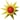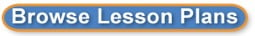Search results for y-intercept:
 Browse All Lesson Plans Lesson Plan Name Grades Interpret the equation 8 to 8(0 stars, 1 ratings)To interpret the equation of line, students will rotate through three stations. Each station will require the students to interpret the equation but using different techniques. Fitness and Math - It All Adds Up 7 to 8(0 stars, 1 ratings)Combining principles of fitness and mathematics, students will see how math is used in the real world. Manipulating Graphs 7 to 8(0 stars, 1 ratings)This lesson will help students make a connection to the slope-intercept form of a graph, y=mx+b and how it relates to the real world.